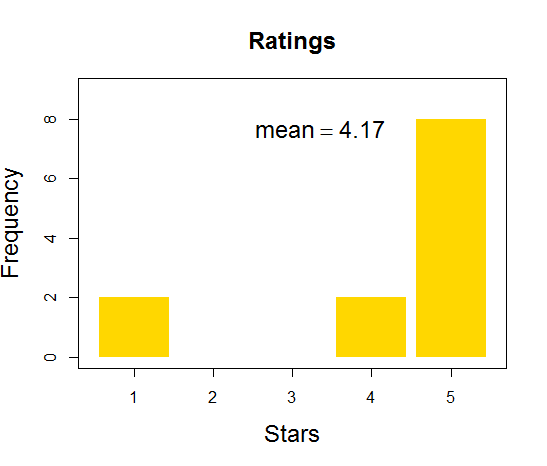## Monday, March 23, 2015

### The impact of outliers on the arithmetic mean (or, do people like this book?)

Consider these ratings of a target item (1 to 5 stars):Based on these ratings, what is your impression of the item? Kinda so-so? Maybe look elsewhere? That's the power of outliers on the arithmetic mean: A few outliers can really pull a mean away from the bulk of the responses. It takes a ton of ratings in the mode to counteract only a few outliers.

These are real data, of course, namely from DBDA2E on Amazon.com. The 1-star ratings have comments that clearly state that they are not rating the content of the book, but still they are 1-star ratings that have a lot of impact on the mean. If you think the mode needs bulking up, you know what to do! :-) And if you have had issues like the 1-star raters have had, please let me know so we can attempt to rectify any problems. (By the way, go here for a link to a discount on the book.)

In general, how can we analyze data that have outliers? One way is describing the data by using a heavy-tailed distribution, which DBDA2E explains extensively in Chapters 16 and 17 (and ordinal data analysis is treated in Chapter 23).

BTW, here's the R code I used for making the graph:

x = c(1,2,3,4,5)
y = c(2,0,0,2,8)
plot( x , y , type="h" , lwd=70 , lend=1 , col="gold" , xlab="Stars" , ylab="Frequency" , main="Ratings" , xlim=c(0.5,5.5) , ylim=c(0,9) , cex.lab=1.5 , cex.main=1.5 )
text( sum(x*y)/sum(y) , max(y) , bquote(mean==.(round(sum(x*y)/sum(y),2))) , adj=c(1,1) , cex=1.5 )